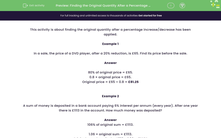# Find the Original Quantity After a Percentage Change

In this worksheet, students will find original quantities after a percentage increase/decrease.Key stage:  KS 3

Curriculum topic:   Number

Curriculum subtopic:   Define, Interpret and Compare Percentages

Difficulty level:#### Worksheet Overview

This activity is about finding the original quantity after a percentage increase/decrease has been applied.

Example 1

In a sale, the price of a DVD player, after a 20% reduction, is £65. Find its price before the sale.

80% of original price = £65.

Divide 80% by 100 to remove the percentage = 0.8

0.8 × original price = £65.

Original price = £65 ÷ 0.8 = £81.25Example 2

A sum of money is deposited in a bank account paying 6% interest per annum (every year). After one year there is £1,113 in the account. How much money was deposited?

106% of original sum = £1,113.

Divide 106 % by 100 to remove the percentage = 1.06

1.06 × original sum = £1,113.

Original sum deposited = £1,113 ÷ 1.06 = £1,050

ALWAYS CHECK that the sale price is less than the original and that an increased quantity is more than the original!If the question is about money, remember to give your answer to 2 decimal places - that is pounds and pence - unless it is a whole number.

Let's get started!

### What is EdPlace?

We're your National Curriculum aligned online education content provider helping each child succeed in English, maths and science from year 1 to GCSE. With an EdPlace account you’ll be able to track and measure progress, helping each child achieve their best. We build confidence and attainment by personalising each child’s learning at a level that suits them.

Get started